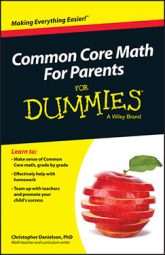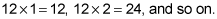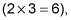##### Common Core Math For Parents For Dummies with Videos OnlineIn fourth grade math, Common Core students begin to study how numbers are built from multiplication relationships. The important relationship here is between factors and multiples.

A number's factors are all the whole numbers by which you can divide it with no remainder. For example, the factors of 12 are 1, 2, 3, 4, 6, and 12. Factors come in pairs, so the factor pairs of 12 are 1 and 12, 2 and 6, and 3 and 4. Sometimes thinking only about the factors that are less than the number in question is useful. They're called proper factors. The proper factors of 12 are 1, 2, 3, 4, and 6.

Meanwhile, the multiples of a number are all the numbers that it divides with no remainder. The multiples of 12 are 12, 24, 36, 48, and so on. Multiplying 12 by any whole number gives a multiple of 12Every number has a limited number of factors and infinitely many multiples.

The key building blocks of numbers are prime numbers. A prime number is a number that has exactly two factors: 1 and itself. For example, 7 is a prime number. The only factors of 7 are 1 and 7. (You can divide 7 by 2, but the answer isn't a whole number.)

Every whole number (besides 1) is either a prime number or it's built out of prime numbers. If a whole number greater than 1 isn't a prime number, it's a composite number. Numbers such as 6, 9, 10, 12, and 100 are examples of composite numbers. For example, 6 has 2 and 3 as a pair of factors,so 6 has more than just 1 and 6 as factors.

In fourth grade, students study the relationship between factors and multiples — namely that a number is a factor of its multiples and is a multiple of its factors. For example, the factors of 6 are 1, 2, 3, and 6. If you list out the multiples of any of these factors, 6 is on that list. The multiples of 2 are 2, 4, 6, 8, and so on. This intimate relationship between factors and multiples often leads to students confusing the terms factor and multiple. But getting the words right every time doesn't matter nearly as much as knowing that numbers can be decomposed with multiplication by using factors.

When a number can be decomposed, this means that you can take the number apart, and you can do it in many ways. For example, you can think of 12 as 2 groups of 6, or as 6 groups of 2, or as 3 groups of 4, and so on.